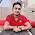# C++ Tutorial With Basic Programs for Beginners

C++ tutorial, C++ programs, C++ code, C++ flowcharts, C++ statements, C++ syntax, C++ examples

## Thursday, January 16, 2014

### Insertion Sort Algorithm Trace Steps and C++ Program

Today, we will discuss Insertion Sort Algorithm Trace Steps on Sample Data and C++ Program implementation of Insertion Sort. First of all, we will:
1. Define Insertion Sort
2. Explain the working of Insertion Sort
3. Design an algorithm of Insertion Sort
4. Show trace steps of Insertion sort algorithm for sample data on paper.
5. Finally, write down the actual code of Insertion sort program in C++.

### What is Insertion Sort?

In Insertion sort, we have to insert elements in to correct position. First of all we find the correct position to insert for the key value under consideration. Secondly we will move other elements to make space for this new element (key value). Now we insert the new element at this space. Suppose there are the following elements in an array A to sort by insertion sort:

### Working of Insertion Sort

1. Let first item be trivially sorted.
2. Now we consider first two items of list. Place second item on its correct location.
3. In Third pass we will consider first three items from list. Insert the 3rd item at its correct location among location1, location2 and location3.
4. In 4th pass, insert the 4th item at its correct location among location1, location2, location3 and location4.
5. And so on.

### Algorithm of Insertion Sort

Algorithm: INSERTION-SORT(array)
This algorithm takes Array 'array' of size n numbers (array has index from 0 to n-1)as input. It sorts the given array in ascending order by INSERTION SORT method. i and j are integer variables used as index of array. temp is a temporary integer variable.
1.   Repeat through step 6 for i ← 1 to n-1
2.     temp  ← array[i]
3.    Repeat through  step 5 while ( j > 0 && array[j-1] > temp)
4.    array[j] ← array[j -1]
5.    j ←   j - 1
6.    array[j]← temp
7.    End

Insertion Algorithm Paper Tracing with data:a ,a, a, a, a, a
5,       2,     4,      6,     1,     3
1. First of all we suppose that the first element 5 is its correct place or trivially sorted.

2. Now we consider second element 2. We have to insert 2 at its correct location from first two locations  and . We will find its correct position from first two items 5 and 2.
3. Since 2 is less than 5, so the correct location of 2 is at array index.
4. We will move 5 from position  to .
5. Now we will place 2 at , so we have sorted first two items.

6. Now array = 2,5,4,6,1,3 Consider 3rd item which is 4. Now we find its correct location from first three locations. So 4 will be inserted at location  between 2 and 5.
7. We move 5 to position  and place 4 on position 
8. So first three items are sorted with array = 2,4,5,6,1,3

9. Now array = 2,4,5,6,1,3   consider 4th item 6 and place on its correct location among first four locations.
We know that 6 is at its correct location which is 4th location and by index it is .

10. Now array is 2,4,5,6,1,3  consider 5th item which is 1. We know that its correct location between first
five items is number one that is position  by index.
11. So we move 6 on 
we move 5 on 
we move 4 on 
we move 2 on 
12. Now place 1 on position , so the array items a to a are sorted

13. Now array is 1,2,4,5,6,3   consider 6th item which is 3.
14. We know that 3 will be inserted at location  between item 2 and item 4.
15. We move 6 to location 
move 5 to 
move 4 to 
16. Now place 3 at location 
17. Hence the whole array has been sorted now     1,2,3,4,5,6

You can check this all process in the following picture of insertion sort paper tracing.

### Insertion Sort Algorithm Trace Steps and Program

Image: Trace steps : Insertion Sort Algorithm Trace Steps and ProgramEasyway C++ : Insertion sort Algorithm trace steps and C++ Program

### Program Insertion Sort in C++

/*
(c)EasyCppProgramming.Blogspot.Com
C++ is Simplified Here!
Target 1001 C++ Example Programs!
EasyCppProgramming.Blogspot.Com
*/

// Program To Input an array and sort
// the array by Insertion Sort Algorithm

#include<iostream>
#include<conio.h>

int main()
{

int n, array, i, j, t;

cout<<"\n/* Insertion sort Ascending order */\n";

cout<<"Enter number of elements\n";
cin>>n;

cout<<"Enter "<<n<<" numbers\n";

for (i = 0; i < n; i++)
cin>>array[i];

for( i = 1;i < n; i++)
{
temp = array[i];
for ( j = i; j > 0 && array[j-1] > temp; j--)
array[j] = array[j-1];
array[j] = temp;
}

cout<<"Sorted list in ascending order:\n";

for (i = 0; i <= n - 1; i++)
cout<<array[i]<<endl;

getch();
return 0;
}

#### Image: Sample Run of Program in Turbo C++ 3.0 - Insertion Sort Algorithm Trace Steps and ProgramEasyway C++ : Insertion sort algorithm C++ Program Output Trace output

### Another Insertion Sorting Example for sorting data: 5,2,3,8,1

Figure: ample data trace steps: Insertion Sort Algorithm Trace Steps and ProgramInsertion Sort Algorithm Trace Steps and Program - easyway C++ Program
So, we have completely discussed Insertion Sort Algorithm Trace Steps and Program, if you have any question please do not hesitate to ask question in comments section, thanks.

Further suggested Reading: Sorting Algorithms and Programs in C / C++

Share:

#### 1 comment:

1.Insertion Sort in C

Insertion Sort is a simplest array data or data Sorting algorithm which sorts the array elements by shifting elements one by one and inserting each element into its proper position.

We Love To Hear From You!